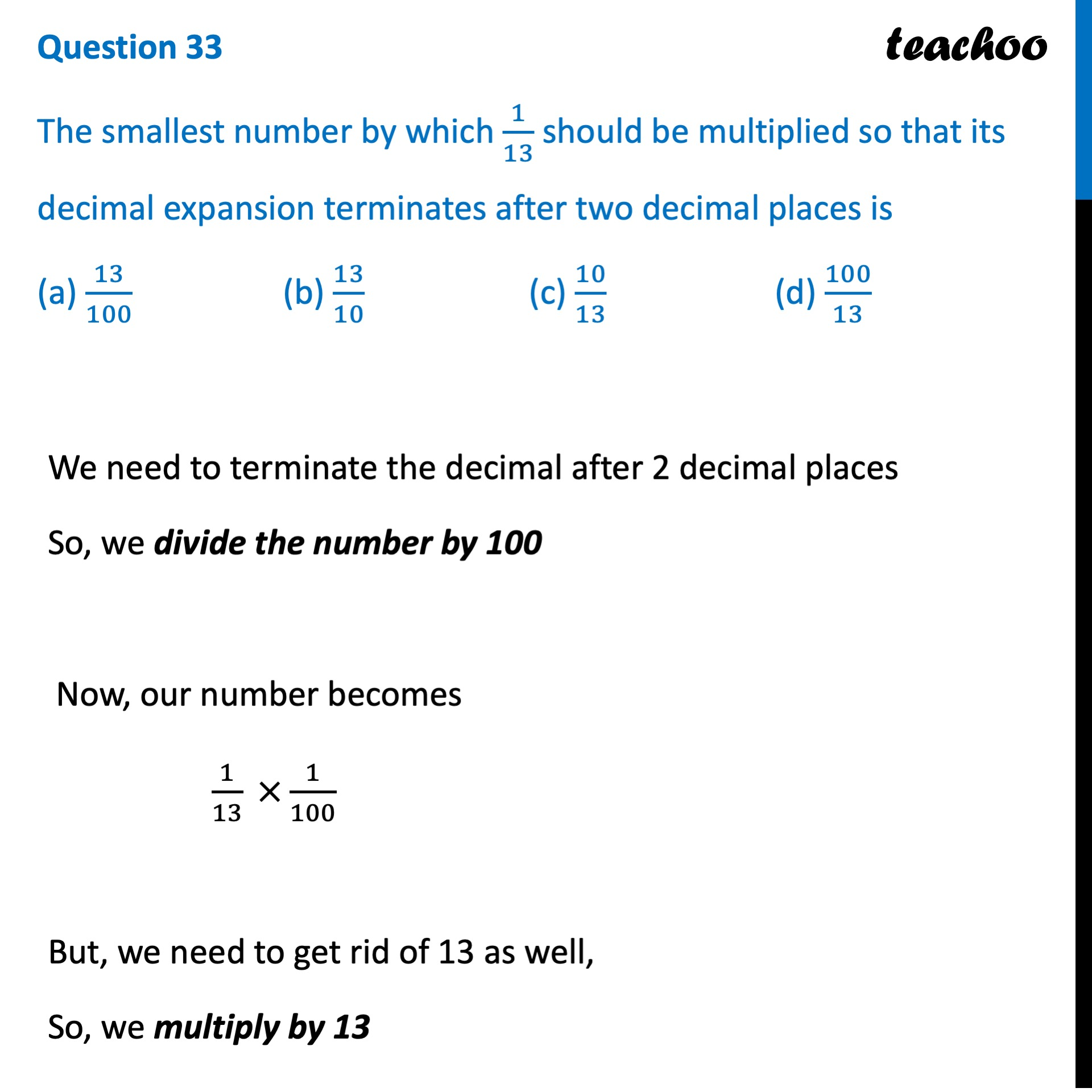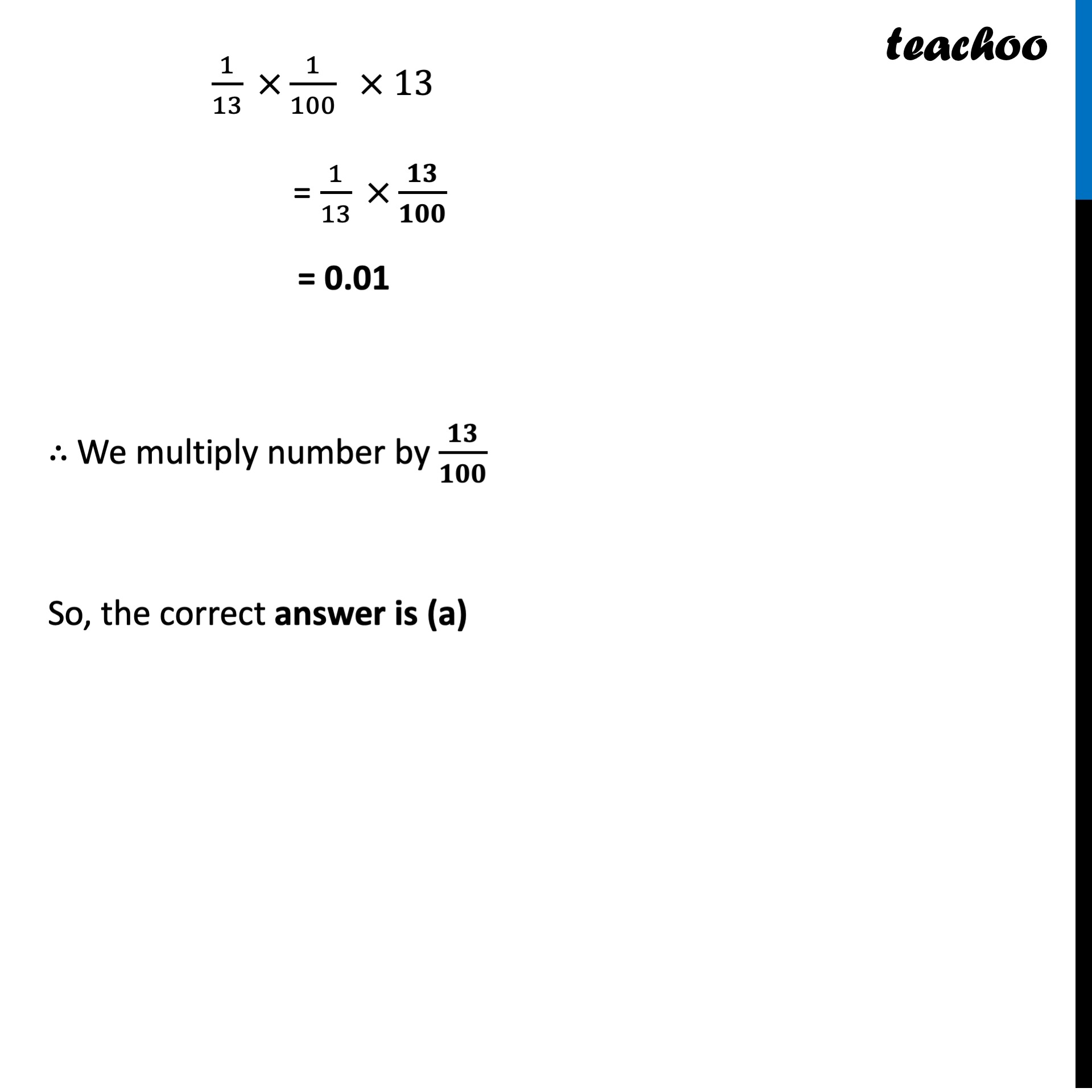CBSE Class 10 Sample Paper for 2022 Boards - Maths Standard [MCQ]

Class 10
Solutions of Sample Papers for Class 10 Boards

## (a) 13/100  (b) 13/10    (c) 10/13   (d) 100/13Learn in your speed, with individual attention - Teachoo Maths 1-on-1 Class

### Transcript

Question 33 The smallest number by which 1/13 should be multiplied so that its decimal expansion terminates after two decimal places is (a) 13/100 (b) 13/10 (c) 10/13 (d) 100/13 We need to terminate the decimal after 2 decimal places So, we divide the number by 100 Now, our number becomes 1/13 ×1/100 But, we need to get rid of 13 as well, So, we multiply by 13 1/13 ×1/100 × 13 = 1/13 ×𝟏𝟑/𝟏𝟎𝟎 = 0.01 ∴ We multiply number by 𝟏𝟑/𝟏𝟎𝟎 So, the correct answer is (a)